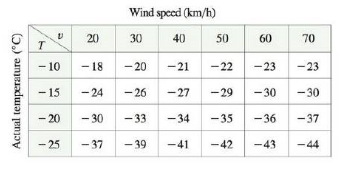Chapter 14.4, Problem 24E

Chapter
Section
Textbook Problem

The wind-chill index W is the perceived temperature when the actual temperature is T and the wind speed is v, so we can write W = f(T, v). The following table of values is an excerpt from Table 1 in Section 14.1. Use the table to find a linear approximation to the wind-chill index function when T is near − 15°C and v is near 50 km/h. Then estimate the wind-chill index when the temperature is −17°C and the wind speed is 55 km/h.To determine

To find: The linear approximation of the wind-chill index function W=f(T,v) at actual temperature near 15C and the wind speed is near 50km/h , use this approximation and approximate the value of f(T,v)=f(17,55) .

Explanation

Given:

The function is, W=f(T,v) where T is actual temperature, v is wind speed.

Calculation:

The linear approximation of the function W=f(T,v) at (15,50) is defined as,

f(15,50)f(15,50)+fT(15,50)(T+15)+fv(15,50)(v50) . (1)

The value of fT(15,50) is defined as, fT(15,50)=limh0f(15+h,50)f(15,50)h

Let h=5 in the above equation,

fT(15,50)=limh0f(15+5,50)f(15,50)5=limh0f(10,50)f(15,50)5=22+295=1.4

Let h=5 and find fT(15,50) ,

fT(15,50)=limh0f(155,50)f(15,50)5=limh0f(20,50)f(15,50)5=27+295=1.2

Compute the average value of, fT(15,50)=1.4 and fT(15,50)=1.2 .

fT(15,50)=1.4+1.22=2.62=1.3

Thus, the value of fT(15,50)=1.3 .

Similarly, the function fv(15,50) is defined as, fv(15,50)=limh0f(15,50+h)f(15,50)h .

Let h=10 and use table to find fv(15,50) .

fv(15,50)=limh0f(15,50+10)f(15,50)10=limh0f(15,60)f(15,50)10=30+2910=0

Still sussing out bartleby?

Check out a sample textbook solution.

See a sample solution

The Solution to Your Study Problems

Bartleby provides explanations to thousands of textbook problems written by our experts, many with advanced degrees!

Get Started

Convert the expressions in Exercises 6584 to power form. 3

Finite Mathematics and Applied Calculus (MindTap Course List)

let f(x) = x3 + 5, g(x) = x2 2, and h(x) = 2x + 4. Find the rule for each function. 6. fgh

Applied Calculus for the Managerial, Life, and Social Sciences: A Brief Approach

For Problems 11-18, evaluate each expression. 44211102

Mathematical Applications for the Management, Life, and Social Sciences